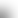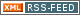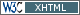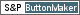www.LinuxHowtos.org howtos, tips&tricks and tutorials for linuxfrom small one page howto to huge articles all in one place

search text in:

Poll
Which filesystem do you use?

poll results

using iotop to find disk usage hogs

using iotop to find disk usage hogs

words:

887

views:

169651

userrating:

average rating: 1.7 (102 votes) (1=very good 6=terrible)

May 25th. 2007:
Words

486

Views

249251

Workaround and fixes for the current Core Dump Handling vulnerability affected kernels

Workaround and fixes for the current Core Dump Handling vulnerability affected kernels

words:

161

views:

135566

userrating:

average rating: 1.4 (41 votes) (1=very good 6=terrible)

April, 26th. 2006:

You are here: manpages

# EC_GROUP_copy

Section: OpenSSL (3)
Updated: 2017-05-25

## NAME

EC_GROUP_copy, EC_GROUP_dup, EC_GROUP_method_of, EC_GROUP_set_generator, EC_GROUP_get0_generator, EC_GROUP_get_order, EC_GROUP_get_cofactor, EC_GROUP_set_curve_name, EC_GROUP_get_curve_name, EC_GROUP_set_asn1_flag, EC_GROUP_get_asn1_flag, EC_GROUP_set_point_conversion_form, EC_GROUP_get_point_conversion_form, EC_GROUP_get0_seed, EC_GROUP_get_seed_len, EC_GROUP_set_seed, EC_GROUP_get_degree, EC_GROUP_check, EC_GROUP_check_discriminant, EC_GROUP_cmp, EC_GROUP_get_basis_type, EC_GROUP_get_trinomial_basis, EC_GROUP_get_pentanomial_basis - Functions for manipulating EC_GROUP objects.

## SYNOPSIS

``` #include <openssl/ec.h>
#include <openssl/bn.h>

int EC_GROUP_copy(EC_GROUP *dst, const EC_GROUP *src);
EC_GROUP *EC_GROUP_dup(const EC_GROUP *src);

const EC_METHOD *EC_GROUP_method_of(const EC_GROUP *group);

int EC_GROUP_set_generator(EC_GROUP *group, const EC_POINT *generator, const BIGNUM *order, const BIGNUM *cofactor);
const EC_POINT *EC_GROUP_get0_generator(const EC_GROUP *group);

int EC_GROUP_get_order(const EC_GROUP *group, BIGNUM *order, BN_CTX *ctx);
int EC_GROUP_get_cofactor(const EC_GROUP *group, BIGNUM *cofactor, BN_CTX *ctx);

void EC_GROUP_set_curve_name(EC_GROUP *group, int nid);
int EC_GROUP_get_curve_name(const EC_GROUP *group);

void EC_GROUP_set_asn1_flag(EC_GROUP *group, int flag);
int EC_GROUP_get_asn1_flag(const EC_GROUP *group);

void EC_GROUP_set_point_conversion_form(EC_GROUP *group, point_conversion_form_t form);
point_conversion_form_t EC_GROUP_get_point_conversion_form(const EC_GROUP *);

unsigned char *EC_GROUP_get0_seed(const EC_GROUP *x);
size_t EC_GROUP_get_seed_len(const EC_GROUP *);
size_t EC_GROUP_set_seed(EC_GROUP *, const unsigned char *, size_t len);

int EC_GROUP_get_degree(const EC_GROUP *group);

int EC_GROUP_check(const EC_GROUP *group, BN_CTX *ctx);

int EC_GROUP_check_discriminant(const EC_GROUP *group, BN_CTX *ctx);

int EC_GROUP_cmp(const EC_GROUP *a, const EC_GROUP *b, BN_CTX *ctx);

int EC_GROUP_get_basis_type(const EC_GROUP *);
int EC_GROUP_get_trinomial_basis(const EC_GROUP *, unsigned int *k);
int EC_GROUP_get_pentanomial_basis(const EC_GROUP *, unsigned int *k1,
unsigned int *k2, unsigned int *k3);

```

## DESCRIPTION

EC_GROUP_copy copies the curve src into dst. Both src and dst must use the same EC_METHOD.

EC_GROUP_dup creates a new EC_GROUP object and copies the content from src to the newly created EC_GROUP object.

EC_GROUP_method_of obtains the EC_METHOD of group.

EC_GROUP_set_generator sets curve paramaters that must be agreed by all participants using the curve. These paramaters include the generator, the order and the cofactor. The generator is a well defined point on the curve chosen for cryptographic operations. Integers used for point multiplications will be between 0 and n-1 where n is the order. The order multipied by the cofactor gives the number of points on the curve.

EC_GROUP_get0_generator returns the generator for the identified group.

The functions EC_GROUP_get_order and EC_GROUP_get_cofactor populate the provided order and cofactor parameters with the respective order and cofactors for the group.

The functions EC_GROUP_set_curve_name and EC_GROUP_get_curve_name, set and get the NID for the curve respectively (see EC_GROUP_new(3)). If a curve does not have a NID associated with it, then EC_GROUP_get_curve_name will return 0.

The asn1_flag value on a curve is used to determine whether there is a specific ASN1 OID to describe the curve or not. If the asn1_flag is 1 then this is a named curve with an associated ASN1 OID. If not then asn1_flag is 0. The functions EC_GROUP_get_asn1_flag and EC_GROUP_set_asn1_flag get and set the status of the asn1_flag for the curve. If set then the curve_name must also be set.

The point_coversion_form for a curve controls how EC_POINT data is encoded as ASN1 as defined in X9.62 (ECDSA). point_conversion_form_t is an enum defined as follows:

``` typedef enum {
/** the point is encoded as z||x, where the octet z specifies
*   which solution of the quadratic equation y is  */
POINT_CONVERSION_COMPRESSED = 2,
/** the point is encoded as z||x||y, where z is the octet 0x02  */
POINT_CONVERSION_UNCOMPRESSED = 4,
/** the point is encoded as z||x||y, where the octet z specifies
*  which solution of the quadratic equation y is  */
POINT_CONVERSION_HYBRID = 6
} point_conversion_form_t;

```

For POINT_CONVERSION_UNCOMPRESSED the point is encoded as an octet signifying the UNCOMPRESSED form has been used followed by the octets for x, followed by the octets for y.

For any given x co-ordinate for a point on a curve it is possible to derive two possible y values. For POINT_CONVERSION_COMPRESSED the point is encoded as an octet signifying that the COMPRESSED form has been used AND which of the two possible solutions for y has been used, followed by the octets for x.

For POINT_CONVERSION_HYBRID the point is encoded as an octet signifying the HYBRID form has been used AND which of the two possible solutions for y has been used, followed by the octets for x, followed by the octets for y.

The functions EC_GROUP_set_point_conversion_form and EC_GROUP_get_point_conversion_form set and get the point_conversion_form for the curve respectively.

ANSI X9.62 (ECDSA standard) defines a method of generating the curve parameter b from a random number. This provides advantages in that a parameter obtained in this way is highly unlikely to be susceptible to special purpose attacks, or have any trapdoors in it. If the seed is present for a curve then the b parameter was generated in a verifiable fashion using that seed. The OpenSSL EC library does not use this seed value but does enable you to inspect it using EC_GROUP_get0_seed. This returns a pointer to a memory block containing the seed that was used. The length of the memory block can be obtained using EC_GROUP_get_seed_len. A number of the builtin curves within the library provide seed values that can be obtained. It is also possible to set a custom seed using EC_GROUP_set_seed and passing a pointer to a memory block, along with the length of the seed. Again, the EC library will not use this seed value, although it will be preserved in any ASN1 based communications.

EC_GROUP_get_degree gets the degree of the field. For Fp fields this will be the number of bits in p. For F2^m fields this will be the value m.

The function EC_GROUP_check_discriminant calculates the discriminant for the curve and verifies that it is valid. For a curve defined over Fp the discriminant is given by the formula 4*a^3 + 27*b^2 whilst for F2^m curves the discriminant is simply b. In either case for the curve to be valid the discriminant must be non zero.

The function EC_GROUP_check performs a number of checks on a curve to verify that it is valid. Checks performed include verifying that the discriminant is non zero; that a generator has been defined; that the generator is on the curve and has the correct order.

EC_GROUP_cmp compares a and b to determine whether they represent the same curve or not.

The functions EC_GROUP_get_basis_type, EC_GROUP_get_trinomial_basis and EC_GROUP_get_pentanomial_basis should only be called for curves defined over an F2^m field. Addition and multiplication operations within an F2^m field are performed using an irreducible polynomial function f(x). This function is either a trinomial of the form:

f(x) = x^m + x^k + 1 with m > k >= 1

or a pentanomial of the form:

f(x) = x^m + x^k3 + x^k2 + x^k1 + 1 with m > k3 > k2 > k1 >= 1

The function EC_GROUP_get_basis_type returns a NID identifying whether a trinomial or pentanomial is in use for the field. The function EC_GROUP_get_trinomial_basis must only be called where f(x) is of the trinomial form, and returns the value of k. Similary the function EC_GROUP_get_pentanomial_basis must only be called where f(x) is of the pentanomial form, and returns the values of k1, k2 and k3 respectively.

## RETURN VALUES

The following functions return 1 on success or 0 on error: EC_GROUP_copy, EC_GROUP_set_generator, EC_GROUP_check, EC_GROUP_check_discriminant, EC_GROUP_get_trinomial_basis and EC_GROUP_get_pentanomial_basis.

EC_GROUP_dup returns a pointer to the duplicated curve, or NULL on error.

EC_GROUP_method_of returns the EC_METHOD implementation in use for the given curve or NULL on error.

EC_GROUP_get0_generator returns the generator for the given curve or NULL on error.

EC_GROUP_get_order, EC_GROUP_get_cofactor, EC_GROUP_get_curve_name, EC_GROUP_get_asn1_flag, EC_GROUP_get_point_conversion_form and EC_GROUP_get_degree return the order, cofactor, curve name (NID), ASN1 flag, point_conversion_form and degree for the specified curve respectively. If there is no curve name associated with a curve then EC_GROUP_get_curve_name will return 0.

EC_GROUP_get0_seed returns a pointer to the seed that was used to generate the parameter b, or NULL if the seed is not specified. EC_GROUP_get_seed_len returns the length of the seed or 0 if the seed is not specified.

EC_GROUP_set_seed returns the length of the seed that has been set. If the supplied seed is NULL, or the supplied seed length is 0, the return value will be 1. On error 0 is returned.

EC_GROUP_cmp returns 0 if the curves are equal, 1 if they are not equal, or -1 on error.

EC_GROUP_get_basis_type returns the values NID_X9_62_tpBasis or NID_X9_62_ppBasis (as defined in <openssl/obj_mac.h>) for a trinomial or pentanomial respectively. Alternatively in the event of an error a 0 is returned.

crypto(3), ec(3), EC_GROUP_new(3), EC_POINT_new(3), EC_POINT_add(3), EC_KEY_new(3), EC_GFp_simple_method(3), d2i_ECPKParameters(3)

## Index

NAME
SYNOPSIS
DESCRIPTION
RETURN VALUES||- Powered by- Running on-
Copyright 2004-2020 Sascha Nitsch Unternehmensberatung GmbH::- Level Triple-A Conformance to Web Content Accessibility Guidelines 1.0 -
- Copyright and legal notices -